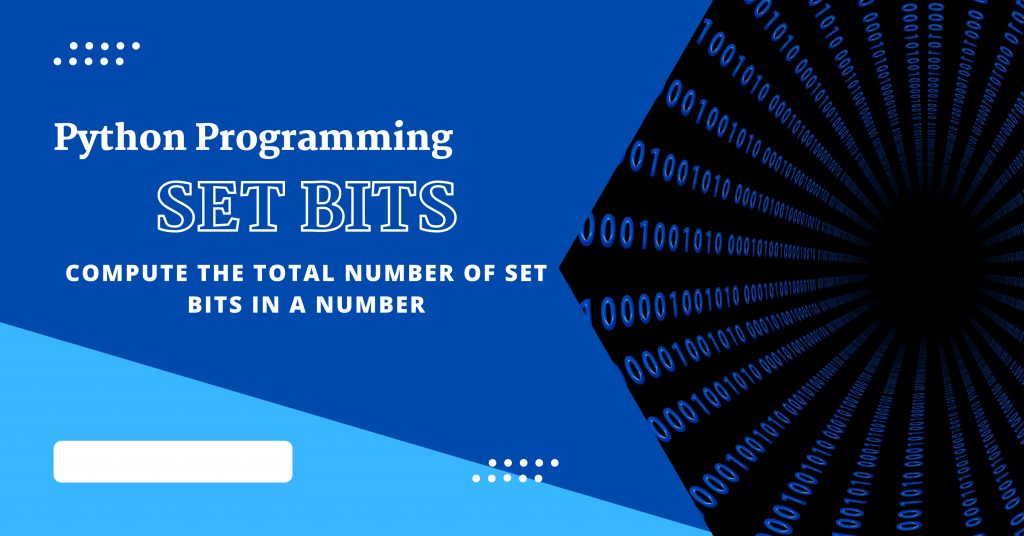# Calculate Set Bits in an Integer in Pythonhey learner! In this tutorial, we will use the Python programming language to calculate the total number of set bits in an integer. This problem will demonstrate the significance and power of the Bit Manipulation concept.

## What are Set Bits – Introduction

In the world of binary numbers, sets bits are represented by 1’s. So, basically, we need to find the total amount of 1’s in a number’s binary form.

## Understanding the problem

Given a number `N`.
Return the total number of sets bits present in the binary version of the number N.

For instance,
If the given number (N) = 5. Then the result is 2 because the binary version of 5 is 101. The total number of 1’s present in the 101 is 2. Hence the number of set bits is 2.

## Approach 1: Manual Conversion

Convert the given decimal number to a binary number and then count the total number of 1s in the converted number’s binary form. However, this is not an efficient solution to the problem.

In this instance, the time complexity will be linear, but we can make this strategy more efficient.

## Approach 2: Bit Manipulation

So, in this approach, we will see one of the Bit Manipulation methods. We can improve the efficiency of our code and approach by employing this method.

So we will be following the steps mentioned below:

1. Check if N>0
2. Compute AND of A and A-1
3. Keep repeating step 2 until A != 0
4. We will keep the count of the no of iterations
5. The count is equal to the number of set bits in the number N

## Calculating Set Bits using Python

```def countsetbits(A):
count = 0
while(A!=0):
A = A & (A-1)
count = count+1
return(count)

n = int(input("Enter the Number: "))
print(countsetbits(n))
```

## Sample Outputs

```Enter the Number: 5
2
```
```Enter the Number: 100
3
```

I hope you understood the concept behind the problem and also the solution to the problem.

Thank you for reading the tutorial! 😇